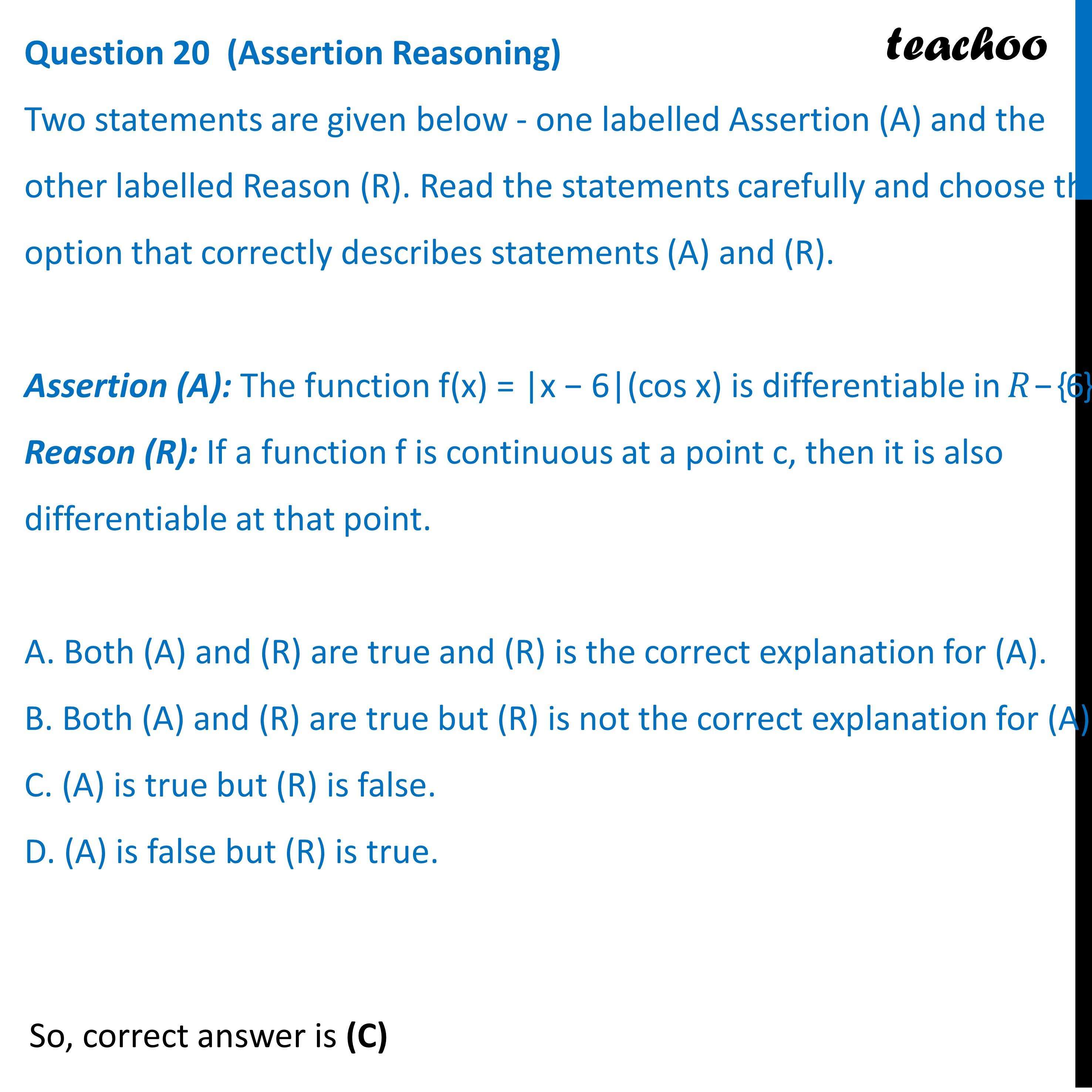Practice Questions CBSE - Maths Class 12 (2023 Boards)

Class 12
Solutions of Sample Papers and Past Year Papers - for Class 12 Boards

## D. (A) is false but (R) is true.Learn in your speed, with individual attention - Teachoo Maths 1-on-1 Class

### Transcript

Two statements are given below - one labelled Assertion (A) and the other labelled Reason (R). Read the statements carefully and choose the option that correctly describes statements (A) and (R). Assertion (A): The function f(x) = |x − 6|(cos x) is differentiable in 𝑅 − {6}. Reason (R): If a function f is continuous at a point c, then it is also differentiable at that point. A. Both (A) and (R) are true and (R) is the correct explanation for (A). B. Both (A) and (R) are true but (R) is not the correct explanation for (A). C. (A) is true but (R) is false. D. (A) is false but (R) is true.